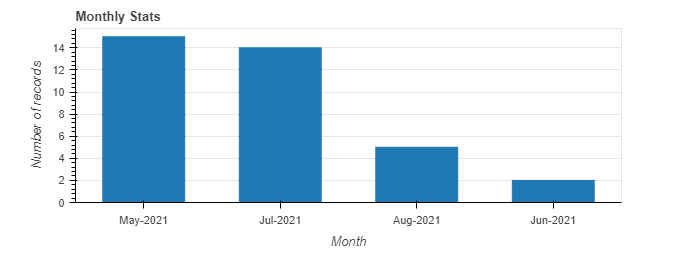Ordering of bars in a bar chart

Hi,

I am new to Bokeh and I was trying the following plot with my pandas df. The df is sorted as per my needs( by chronological order of month) and I want the bars to appear in the same way(May,June,July etc…,) while the bars shown are in the descending order of value for each month. Is there a way to sort the bars according to the df ?
This is my sample code snippet.

data=mdf

p = figure(x_range=mdf[‘month’], plot_height=250, title=“Monthly Stats”,
toolbar_location=None, tools="")

p.vbar(x=mdf[‘month’], top=mdf[‘count’], width=0.6)
p.xgrid.grid_line_color = None
p.xaxis.axis_label = “Month”
p.yaxis.axis_label = “Number of records”
p.y_range.start = 0
show(p)Thanks,
Ananya

Three categories appear in exactly the order you specify in x_range

Handling categorical data — Bokeh 2.4.2 Documentation

This topic was automatically closed 90 days after the last reply. New replies are no longer allowed.